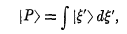# Eigenkets belonging to a range of eigenvalues

• I
• Spin One
In summary, when you want to represent a general ket in a basis consisting of eigenkets each attributed to an eigenvalue in a range, you take the integral of said kets from a to b w.r.t. the eigenvalues. This formalizes the notion of a limit as the sum of a series of individual terms. If the coefficients of the eigenkets change slowly with respect to the eigenvalues, then you can approximate the kets by a continuous basis with a different normalization.

#### Spin One

When one wants to represent a general ket in a basis consisting of eigenkets each attributed to an eigenvalue in a range, say from a to b, why does one take the integral of said kets from a to b w.r.t. the eigenvalues?I understand that the integral here plays a role analogous to a sum in the case where a general ket is expressed in terms of eigenkets belonging to discrete eigenvalues, but I don't understand why each vector is multiplied by an infinitesimal change near the eigenvalue it belongs to. Interpreting this integral as the limit of a sum we get:
[P>=Σ[ξ>Δξ (lim.Δξ→0)
where I do not understand the role of Δξ.

#### Attachments

I looked over the thread about Rigged Hilbert Spaces, and I'm not sure that it completely explained the relationship between continuous and discrete bases.

In a non-rigorous way, you can think of the continuous basis as a limiting case of the discrete basis. However, in going from discrete to continuous, the normalization convention for basis elements changes.

Let me illustrate. Suppose you have an operator ##\Lambda## with discrete eigenvalues ##\lambda_j##. I think in order for the continuum limit to make sense int the most straightforward way, you need to assume that ##\lambda_{j+1} > \lambda_j##, and that there are infinitely many ##\lambda_j##, and that the corresponding eigenstates ##|n\rangle## form a complete orthonormal basis. That means that
1. If ##n \neq m##, then ##\langle n|m\rangle = 0##
2. ##\langle n|n\rangle = 1##
3. If ##|\psi\rangle## is a properly normalized state, then ##|\psi\rangle = \sum_n \langle n|\psi\rangle |n\rangle##
4. ##\sum_n |\langle n|\psi\rangle|^2 = 1##
Now, if the coefficients ##\langle n|\psi\rangle## change slowly with ##n## (and maybe we also have to assume that ##(\Delta \lambda)_n \equiv \lambda_{n+1} - \lambda_n## remains bounded? I'm not sure...) then we can define a new ket with a different normalization:

##|\lambda_n\rangle \equiv \frac{1}{\sqrt{(\Delta \lambda)_n}} |n\rangle##

In terms of the ##|\lambda_n\rangle##, we have:

##|\psi\rangle = \sum_n (\Delta \lambda)_n \langle \lambda_n |\psi\rangle |\lambda_n\rangle##

The kets ##|\lambda_n\rangle## have a different normalization:

• ##\langle \lambda_n | \lambda_m \rangle = 0## (if ##m \neq n##)
• ##\langle \lambda_n | \lambda_n \rangle = \frac{1}{(\Delta \lambda)_n}##
If the states ##|\lambda_n\rangle## change smoothly with ##n##, then this can be approximated by an integral:

##|\psi\rangle = \int d\lambda \langle \lambda |\psi\rangle |\lambda\rangle##

Last edited:
So dλ is introduced to make the product between each eigenket and dλ finite, since the eigenkets will be of "infinite length" in the sense of lim.Δλ→0[1/Δλ]. That makes sense. In that case, will the coefficients <λIΨ> be infinitesimal? Otherwise the integral would diverge, even over a finite range.

Spin One said:
So dλ is introduced to make the product between each eigenket and dλ finite, since the eigenkets will be of "infinite length" in the sense of lim.Δλ→0[1/Δλ]. That makes sense. In that case, will the coefficients <λIΨ> be infinitesimal? Otherwise the integral would diverge, even over a finite range.

Yes - but unless you want to get into non-standard analysis infinitesimals are a load of the proverbial, although used every now and then when speaking informally. I do it but shouldn't really.

There is no way to understand it properly unless you study the references in my link.